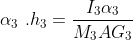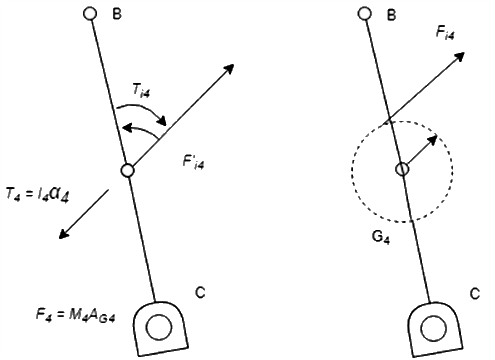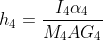INTRODUCTION

Learning Objectives:

1. State the concepts of dynamic analysis of four bar mechanisms
2. Explain the significance of the parameter and select the variables accordingly. Parameters are velocity, angular velocity, acceleration, angular acceleration and forces. Variables are link lengths, mass of links, input angular velocity and torque.
3. Calculate the velocity, acceleration and force acting on each link.
4. Assess and compare velocity, acceleration, acceleration of CG, offset dimensions, force of the calculated value with the simulator.
5. Evaluate how change in length, angle and driving force results in change of acceleration, velocity and work done by the mechanism.

Theory

The dynamic analysis of the four bar mechanism is covered after understanding the kinematic analysis since the acceleration of the links are required to calculate the forces on the link. The analysis involves the offset analysis, acceleration of CG, forces on each link of four bar mechanism, with the help of position diagram, velocity and acceleration diagram.

OABC is a 4–bar mechanism. Link 2 rotates with constant angular velocity ω2.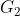,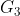&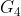are the center of gravity and m2, m3 & m4 the masses of links 2, 3 & 4 respectively.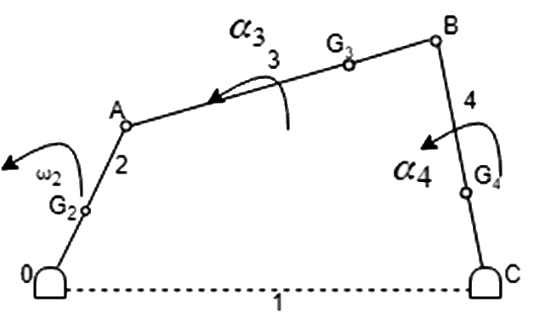1. Draw the velocity & acceleration polygons for determine the linear acceleration of,&2. Magnitude and sense of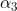&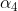(angular acceleration) are determined using the results of step 1.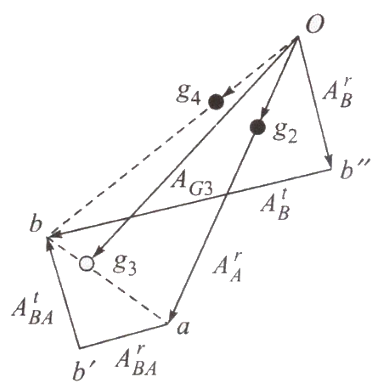To determine inertia forces and couples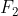= accelerating force (towards O)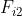= inertia force (away from O)

Since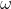is constant,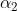= 0 and no Inertia torque involved.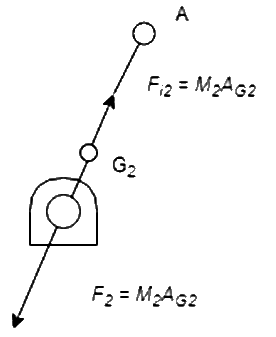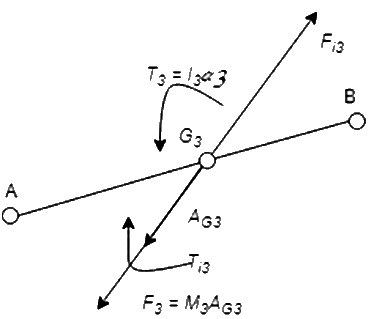Linear acceleration of(i.e.,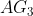is in the direction of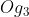of acceleration polygon).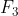= accelerating force

Inertia force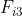acts in opposite direction. Due to, there must be a resultant torque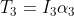acting in the sense of(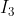is MMI of the link about an axis through, perpendicular to the plane of paper). The inertia torque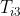is equal and opposite of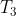.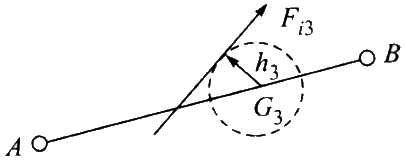can replace the inertia forceand the inertia torque.is tangent to circle of radius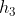fromon the top side of it so as to oppose the angular acceleration